Test: Electricity - 2

# Test: Electricity - 2

Test Description

## 20 Questions MCQ Test Science & Technology for UPSC CSE | Test: Electricity - 2

Test: Electricity - 2 for Class 10 2023 is part of Science & Technology for UPSC CSE preparation. The Test: Electricity - 2 questions and answers have been prepared according to the Class 10 exam syllabus.The Test: Electricity - 2 MCQs are made for Class 10 2023 Exam. Find important definitions, questions, notes, meanings, examples, exercises, MCQs and online tests for Test: Electricity - 2 below.
Solutions of Test: Electricity - 2 questions in English are available as part of our Science & Technology for UPSC CSE for Class 10 & Test: Electricity - 2 solutions in Hindi for Science & Technology for UPSC CSE course. Download more important topics, notes, lectures and mock test series for Class 10 Exam by signing up for free. Attempt Test: Electricity - 2 | 20 questions in 20 minutes | Mock test for Class 10 preparation | Free important questions MCQ to study Science & Technology for UPSC CSE for Class 10 Exam | Download free PDF with solutions
 1 Crore+ students have signed up on EduRev. Have you?
Test: Electricity - 2 - Question 1

### The correct relation between current 'I' and amount of charge flown 'q' during a time 't' through a conductor is

Detailed Solution for Test: Electricity - 2 - Question 1

Correct relation is current I =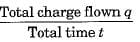⇒ q = It

Test: Electricity - 2 - Question 2

### The effective resistance between A and B is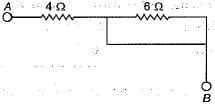Detailed Solution for Test: Electricity - 2 - Question 2

6Ω is shorted so effective resistance is 4Ω.

Test: Electricity - 2 - Question 3

### SI unit of resistivity is

Detailed Solution for Test: Electricity - 2 - Question 3

As resistivity of the material of a resistor of length l, cross-section area A and resistance R is given by :ρ = R.A/I, hence SI unit of resistivity is ohm -metre (Ω m).

Test: Electricity - 2 - Question 4

A cooler of 1500 W, 200 volt and a fan of 500 W, 200 volt are to be used from a household supply. The rating of fuse to be used is

Detailed Solution for Test: Electricity - 2 - Question 4

Total power used, P - P1 + P2 = 1500 + 500 = 2000 W.
Current drawn from the supply,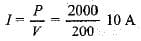Test: Electricity - 2 - Question 5

If four resistors each of 1Ω are connected in parallel, the effective resistance will be

Detailed Solution for Test: Electricity - 2 - Question 5

If four resistors each of 1Ω are connected in parallel, the effective resistance will be 0.25Ω..

As, in parralel connection,

1/Reff. = 1/R1 + 1/R2 + ....

Test: Electricity - 2 - Question 6

A fuse wire repeatedly gets burnt when used with a good heater. It is advised to use a fuse wire of

Detailed Solution for Test: Electricity - 2 - Question 6

In order to get the working of heater properly, fused wire of higher rating must be used.

Test: Electricity - 2 - Question 7

Resistivity of a given conductor depends on

Detailed Solution for Test: Electricity - 2 - Question 7

Resistivity of a given conductor depends only on its material and the temperature. Resistivity does not depend on the dimensions of the conductor.

Test: Electricity - 2 - Question 8

A coil in the heater consume power P on passing current. If it is cut into halves and joined in parallel, it will consume power

Detailed Solution for Test: Electricity - 2 - Question 8

Original power consumed, P = V2/R
When used in parallel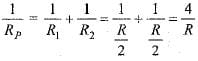RP = R/4
∴ New power consumed when two halves in parallel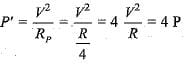Test: Electricity - 2 - Question 9

A combination of three resistances has been shown here. Effective resistance between the points A and B is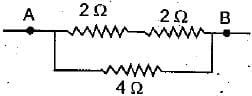Detailed Solution for Test: Electricity - 2 - Question 9

Value of series combination of 2Ω and 2Ω resistances = 4Ω. As it is now connected in parallel to 4Ω resistance, hence the effective resistance R between the points A and B will be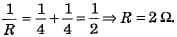Test: Electricity - 2 - Question 10

If P and V are the power and potential of device, the power consumed with a supply potential Vis

Detailed Solution for Test: Electricity - 2 - Question 10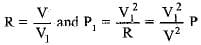Test: Electricity - 2 - Question 11

Which alloy is used to prepare the heating element of an electric iron ?

Detailed Solution for Test: Electricity - 2 - Question 11

An alloy ‘nichrome’ is used to prepare the heating element of an electric iron because its resistivity is high, its melting point is high and it does not oxidise easily.

Test: Electricity - 2 - Question 12

The resistance of hot filament of the bulb is about 10 times the cold resistance. What will be the resistance of 100 W-220 V lamp, when not in use ?

Detailed Solution for Test: Electricity - 2 - Question 12

When the lamp is on use, it is hot.
Using joules law of heating ;
P =  V2 / R
R  =  2202 /100  = 484 ohm.
It is given resistance of hot lamp is 20 times the cold lamp.
Hence, R cold  = 484/10 = 48.4 ohm.

Test: Electricity - 2 - Question 13

Two electric lamps are rated as 220 V, 100 W and 220 V, 40 W respectively. Their electric resistances are

Detailed Solution for Test: Electricity - 2 - Question 13

Resistance R = V2/P where V = voltage/potential difference and P - power
∴ electrical resistance of 100 W lamp,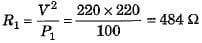and electrical resistance of 40 W lamp,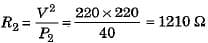Test: Electricity - 2 - Question 14

If R1 and R2 be the resistance of the filament of 40 W and 60 W respectively operating 220 V, then

Detailed Solution for Test: Electricity - 2 - Question 14

Using power,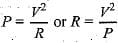For the same voltage, R ∝ 1/P
More the power, lesser the resistance.
Accordingly, R2 < R1

Test: Electricity - 2 - Question 15

A wire of resistance 8Ω is bent in the form of a closed circle. What is the effective resistance between the two points A and B, at the ends of any diameter of the circle ?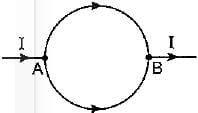Detailed Solution for Test: Electricity - 2 - Question 15

When a wire of 8Ω resistance is bent in the form of a closed circle, resistance of each half (semicircular) part is 8/2 Ω = 4Ω.
As these two parts are connected in parallel across points A and B, hence effective resistance will be 2Ω.

Test: Electricity - 2 - Question 16

Two resistors are connected in series gives an equivalent resistance of 10Ω. When connected in parallel, gives 2.4Ω. Then the individual resistance are

Detailed Solution for Test: Electricity - 2 - Question 16

In series, Rs = R1 + R2 = 1OΩ
In parallel,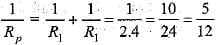Test: Electricity - 2 - Question 17

The rheostat is used in the circuit to :

Detailed Solution for Test: Electricity - 2 - Question 17

A rheostat is used to increase or decrease the magnitude of the current flowing through a circuit without change in the voltage source.

Test: Electricity - 2 - Question 18

Calculate the current flows through the 10Ω resistor in the following circuit.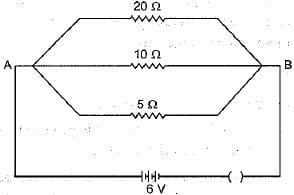Detailed Solution for Test: Electricity - 2 - Question 18

In parallel, potential difference across each resistor will remain same. So, current through 10Ω resistor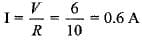Test: Electricity - 2 - Question 19

The graph between current I and potential difference V in the experimental verification of Ohm’s law were drawn by four students. Which one is correct ?

Detailed Solution for Test: Electricity - 2 - Question 19

I-V graph shown in figure (b) is correct because I ∝ 7.

Test: Electricity - 2 - Question 20

Two Wires of same length and area, made of two materials of resistivity ρ1 and ρ2 are connected in parallel V to a source of potential. The equivalent resistivity for the same length and area is

Detailed Solution for Test: Electricity - 2 - Question 20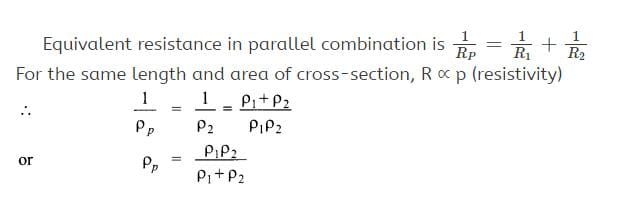## Science & Technology for UPSC CSE

85 videos|355 docs|218 tests
Information about Test: Electricity - 2 Page
In this test you can find the Exam questions for Test: Electricity - 2 solved & explained in the simplest way possible. Besides giving Questions and answers for Test: Electricity - 2, EduRev gives you an ample number of Online tests for practice

## Science & Technology for UPSC CSE

85 videos|355 docs|218 tests(Scan QR code)